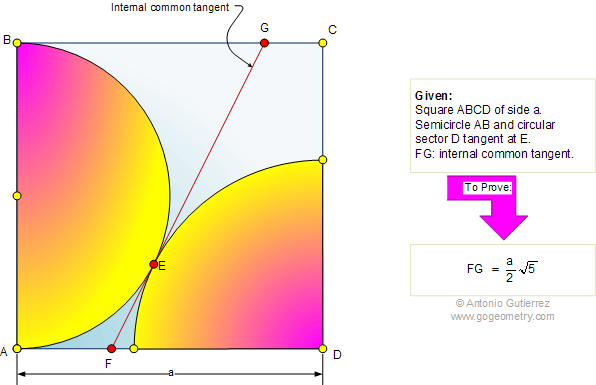# Problem 458: Square, Semicircle, Circular Sector, Internal Common Tangent, Measurement

 The figure shows a square ABCD of side a. The semicircle of diameter AB and the circular sector of center D are tangent at E. If FG is the internal common tangent, prove that. See also: Artwork of Problem 458.More Problems: Problem 458, Artwork. PicSketch for iPhone and iPad, Photo Filter Effects Triangle and altitudes. Proposed Problem 459. Right triangle, Squares, Distance, Measurement. Proposed Problem 457. Triangle, First Brocard Point, Congruent angles, Circle, Circumradius. Proposed Problem 456. Three tangent circles, Center, Chord, Secant, Collinear. Proposed Problem 455. Rhombus, Inscribed Circle, Angle, Chord, 45 Degrees.Home | Search | Geometry | Problems | All Problems | Visual Index | 451-460 | Semicircle | Circle Inscribed in a Square | Email | Post a comment or solution | By Antonio Gutierrez